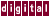# A.5 Data Dependence Direction Vectors

The direction vector is defined as a sequence of direction vector elements (one element for each loop enclosing both statements involved in the dependence arc). The following symbols are direction vector elements: <, =, >, <, >, /, or *. Refer to the following loop:

```     Loops        Line
+---------   10      for ( i=1; i<n ; i++) {
|            11       a[i] = b[i] + c[i];
|            12       c[i] = a[i-1] - 1;
|_________   13       }
```

If line 12 in iteration `I"` depends on line 11 in iteration `I'` , the element of the direction vector for loop `I` is as follows:

```direction
vector
element     when

<    I' must be < I"
=    I' must be = I"
>    I' must be > I"
<=   I' must be < or = I"
>=   I' must be > or = I"
/    I' must not = I"
*    no relation between I' and I" can be proven
```

In the previous example, the dependence for variable a has a direction vector of `<` , because the dependence flows from iteration `I'` to iteration `I'+1` and `I' < I'+1` . For example, the dependence on `a` flows from iteration 1 to iteration 2, and `1 < 2.` The data dependence for the variable `c` has a direction vector of `=` because the dependence stays in the same iteration of the loop (from iteration `I'` to `iteration I'` ).

Previous Page Next Page Contents Index
Command-Line Qualifiers